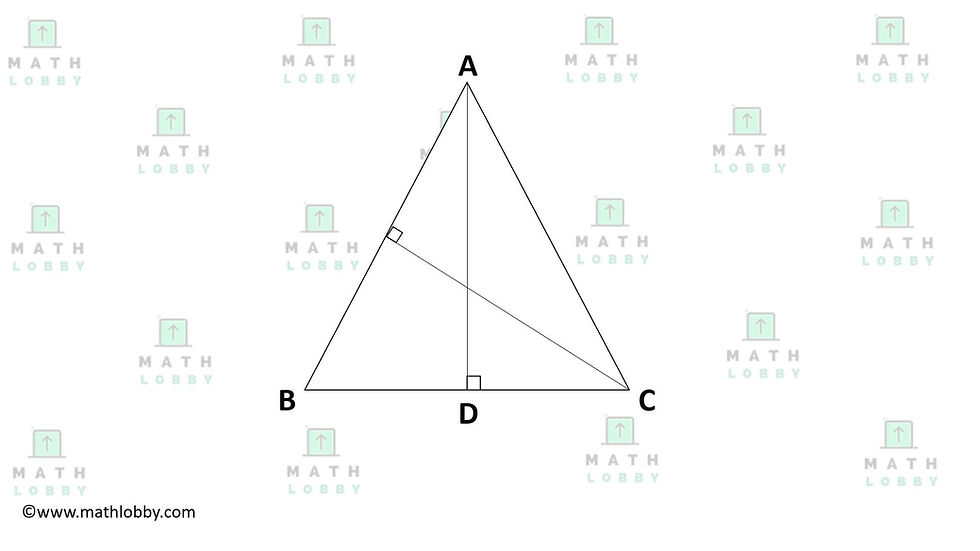top of page
Search

# Triangles in Geometry

Updated: Jun 22, 2021

Dear Secondary Math students, Math Lobby will be going through the topic Triangles in Geometry today. Let's begin!

In this note, you will learn:

1) Types of triangles (Isosceles, equilateral, acute, right and obtuse-angled)

2) Properties of triangles

### 1) Types of triangles (Isosceles, equilateral, acute, right and obtuse-angled)

In geometry, we often encounter a three-sided shape: triangles. However, do you know that there are a number of orientations a triangle can be in which renders it to have different unique characteristics? Let’s take a look at the types of triangles below:There are essentially five types of triangles which we will be focusing on since there have individual unique characteristics to them:

Isosceles triangles – which has two sides of equal length, and the angles opposite each side is equal as well.

Equilateral triangles – which has all three sides of equal length and the angles of all the three sides are equal at 60° each.

Acute-angled triangles – which has all three of its angles to be less than 90° each.

Right-angled triangles – which has one of the three angles to be 90°.

Obtuse-angled triangles – which has one of the three angles to be greater than 90°.

Question time!Can you identify the different types of triangles in the diagram above? Leave your answers in the comments down below!

### Properties of a triangles

Apart from the unique characteristics that the triangles possess individually, there are some properties of a triangle that applies to each and every type of triangle, regardless of the length of its side or angle.

These properties are:

- The sum of all the angles in a triangle is always equal to 180°.

- The side opposite the greater angle is the longest side of all the three sides of a triangle.

- The exterior angle of a triangle is always equal to the sum of the interior opposite angles, which is also known as the exterior angle property.

- Two triangles are said to be similar if their corresponding angles are congruent and lengths of their sides are proportional (More on this in the articles, “Congruent Triangles” and “Similar Triangles”, check them out!)

By definition, area of a triangle is the region occupied by a triangle in a two-dimensional plane, with the SI unit of square unit (or unit²) and its formula is defined by:

Area of a triangle = ½ x base x height

On the other hand, perimeter of a triangle is the length of the outline surrounding the triangle, hence to find the perimeter of a triangle, just simply add up the sides of the triangle!

Perimeter of a triangle = Sum of all the three sides of the triangle

Question Time!

1. Find the area of the triangle when

a) Base = 5m, Height = 10m

b) Base = 50cm, Height = 2m

c) Base = 15m, Height = 0.1km

2. Find the height of the triangle when

a) Area = 300m2, Base = 24m

b) Area = 50m2, Base = 2.9m

c) Area = 24.8m2, Base = 3.5m

3. The three sides of a triangle have the ratio 3: 5: 7. The perimeter of the triangle is known to be 150m. Find the area of the triangle.

*Hint: Pythagoras’ Theorem (If you are not familiar with the concept of Pythagoras’ Theorem, we have an article on this particular topic, “Pythagoras’ Theorem”, check it out!)4. Triangle ABC is isosceles with AB = AC = 10cm, BC = 7cm. The height AD from A to BC is 9cm. Find the area of triangle ABC and therefore, the height from C to AB?

And that’s all we have for today, students! Math Lobby hopes that after this article, all of you would have a better understanding of the numerous types of triangles that exists and its unique properties which are beautiful discoveries in the world of mathematics!

If you have any questions or queries, please feel free to contact Math Lobby on our Facebook page, Instagram or on the Math Lobby website itself!

As always: Work hard, stay motivated and we wish all students a successful and enjoyable journey with Math Lobby!

If you want to receive daily Secondary Math Tips from us,

FOLLOW our Instagram page at https://www.instagram.com/mathlobbymotivation/

*

*

*